## Matlab scripts for Model I and Model II regressions

#### A basic introduction to Model I and Model II linear regressions:

• What they are,
• How they are different,
• Why they are different,
• And when to use them.

#### A brief history of Model II regressions.

• Who led the intellectual development of these regression techniques.
• Plus, a list of their seminal papers.

• Model I regressions: normal (Y-on-X), reversed (X-on-Y) and weighted (wY-on-X).
• Model II regressions: major axis, geometric mean and least-squares-cubic.
• Summary of modifications made to these files.

#### Some rules of thumb to help decide which model regression to use:

• When to use Model I vs Model II.
• Within each type, which of the various models to use.

#### Testing Model I and Model II regressions:

• Evaluate the Model I linear regressions using data from Bevington and Robinson (2003)
• Examine the results for standard and weighted regressions.
• Download the data files – Table 6.1 and Table 6.2ASCII text file format
• Compare both linear regression models.
• Examine the results from the various regression models.
• View graphs of the regression models.
• How to know which regression you are using.

#### For further reading regarding Model I and II regressions, see:

• Ricker (1973). Linear regressions in Fishery Research. J. Fish. Res. Board Can. 30: 409-434.
• Laws and Archie (1981). Appropriate use of regression analysis in marine biology. Marine Biology 65: 13-16.
• Bevington & Robinson (1992). Data Reduction and Error Analysis for the Physical Sciences, Second Edition, McGraw-Hill, Inc., New York.
• Sokal and Rohlf (1995). Biometry, 3rd edition. W.H. Freeman and Company, San Francisco, CA.
• Laws (1997). Mathematical Methods for Oceanographers. John Wiley and Sons, Inc., New York, NY.
• Bevington & Robinson (2003). Data Reduction and Error Analysis for the Physical Sciences, Third Edition, McGraw-Hill, Inc., New York.

#### What are linear regressions?

• Linear regression is a statistical method for determining the slope and intercept parameters for the equation of a line that “best fits” a set of data.
• The most common method for determining the “best fit” is to run a line through the centroid of the data (see below) and adjust the slope of the line such that the sum of the squares of the offsets between the line and the data is a minimum. Thus, this is called the “least squares” technique.
• The centroid is the point determined by the mean of the x-values and the mean of the y-values. Regardless of the model chosen, the line will pass through the centroid of the data. For weighted data sets, the line will pass through the weighted centroid.
• Among the various models, there are different methods for calculating the offsets between the line and the data points. Since many of these methods minimize the sum of the squares of the offsets, they are all called “least squares” techniques; because of this, the term “least squares” does not designate a specific nor a unique method.

#### How are Model I and Model II regressions different?

• In the case of Model I regressions, the offsets are measured parallel to one of the axes. For example, in the regression of Y-on-X (the most common regression technique), this would be parallel to the Y-axis. So, we fit the line by minimizing the sum of the squares of the y-offsets. For the X-on-Y regression, we would use the x-offsets measured parallel to the X-axis.
• For Model II regressions, the offsets are measured along a line perpendicular (or normal) to the regression line. Thus, to use Pearson’s term, the line is fit by minimizing the sum of the squares of the normal deviates.

#### Why are Model I and Model II regressions different?

• In the case of Model I regressions, X is the INDEPENDENT variable and Y is the DEPENDENT variable: X is frequently controlled by the experimenter (or known very precisely) and Y varies in response to the changes in X. One assumes little or no error in X and all regression error is attributed to measurement or other error in Y. The equation tells us how Y varies in response to changes in X.
• For Model II regressions, neither X nor Y is an INDEPENDENT variable but both are assumed to be DEPENDENT on some other parameter which is often unknown. Neither are “controlled”, both are measured, and both include some error. We do not seek an equation of how Y varies in response to a change in X, but rather we look for how they both co-vary in time or space in response to some other variable or process. There are several possible Model II regressions. Which one is used depends upon the specifics of the case. See Ricker (1973) or Sokal and Rohlf (1995, pp. 541-549) for a discussion of which may apply. For convenience, I have also compiled some rules of thumb.
• Karl Pearson  was the “first” to address the problem of fitting a line when both the X and Y variables had measurement error. He called his solution to the problem the “major axis” of the data ellipse. It describes how X and Y co-vary.
• Kermack and Haldane  later showed that when the units of the X and Y variables were changed the major axis was not uniquely determined: the slope and intercept would vary (even after correction for the new axes) when the scales were changed. They proposed the use of a “reduced major axis” where both X and Y were converted to standardized variables. For standardized variables, the mean = 0 and the standard deviation = 1.
• York  developed a method of weighing the data points in both X and Y for those cases where one wants to find the major axis but the uncertainties of the two measurements are different. He called his method the least squares cubic because it requires the solution of a cubic equation to find the slope of the regression, not because it gives a cubic equation.
• Ricker  showed the the geometric mean regression is identical to the reduced major axis but far easier to compute.
• Jolicoeur  took great exception to some of Ricker’s comments; most notably:
• he pointed out that “ l’axe majeur des variables reduites” is more accurately translated standard major axisrather than reduced major axis which Kermack and Haldane  had translated literally from the French;
• that formulae for the asymmetrical confidence limits for the slope of the geometrical mean regression already existed;
• and, that because of the lack of sensitivity of the slope of the standard major axis to the strength of the relationship, the use of the bivariate structural relationship was preferred.
• In his reply, Ricker  took great pains to address each of Jolicoeur’s complaints:
• he presented the formulae for the asymmetrical confidence limits for the slope of the geometrical mean regression and agreed that they provided better limits than his approximate symmetrical limits. However, because of their computational complexity, I have not included them here.
• and, he robustly defended the geometric mean regression as always suitable for naturally variable data.
• Subsequently, Sprent and Dolby  took exception to the ad hoc use of the geometric mean regression in model II cases. They argue that an equally strong case can be made for the line that bisects the minor angle between the two model I regressions: Y-on-X and X-on-Y. (Let’s call this line the least squares bisector.) While the differences in slope between the geometric mean regression and the least squares bisector are small and probably not statistically significant, this new “regression” line is included here for the sake of completeness.
• Even so, Ricker  has been extensively cited, including:
• Laws’ and Archie’s  presentation of a very illustrative (biological) example of the pitfalls of using the model-I regression when a model-II regression is required.
• Sokal’s and Rohlf’s  textbook “Biometry” where the issues of model-I vs model-II regression are discussed in great detail.
• Recently, Laws  has written a book which contains a collection of mathematical and statistical methods commonly used by oceanographers. It includes an extensive chapter where various aspects of model-II regression techniques are presented.
• Bevington and Robinson  have an excellent chapter in their book that describes the derivation of several of the model I regression lines and the statistics used to calculate the slope and y-intercept plus their standard derivations. In a later chapter, they also describe the derivation of the correlation coefficient, r, and how it is calculated.
• And, finally, York et al.  have derived unified equations for the slope, intercept and standard errors of the best straight line for model-II cases showing that the least-squares estimation (LSE) and maximum likelihood estimation (MLE) methods yield identical results. Furthermore, they show that all known correct regression solutions in the literature can be derived from the original York equations .

### References

1. Pearson (1901). On lines and planes of closest fit to systems of points in space. Phil. Mag. v2(6): 559-572.
2. Kermack & Haldane (1950). Organic correlation and allometry. Biometrika v37: 30-41.
3. York (1966). Least-squares fitting of a straight line. Canad. J. Phys. 44: 1079-1086.
4. Ricker (1973). Linear regressions in Fishery Research. J. Fish. Res. Board Can. 30: 409-434.
5. Jolicoeur (1975). Linear Regressions in Fishery Research: Some Comments. J. Fish. Res. Board Can. 32: 1491-1494.
6. Ricker (1975). A Note Concerning Professor Jolicoeur’s Comments. J. Fish. Res. Board Can. 32: 1494-1498.
7. Sprent and Dolby (1980). The Geometric Mean Functional Relationship. Biometrics 36: 547-550.
8. Laws and Archie (1981). Appropriate use of regression analysis in marine biology. Mar. Biol. 65: 13-16.
9. Sokal and Rohlf (1995). Biometry, 3rd edition. W. H. Freeman and Company, San Francisco, CA.
10. Laws (1997). Mathematical Methods for Oceanographers. John Wiley and Sons, Inc., New York, NY.
11. Bevington and Robinson (2003). Data reduction and error analysis for the physical sciences. 3rd edition. McGraw-Hill, New York, NY.
12. York et al. (2004). Unified equations for the slope, intercept, and standard errors of the best straight line. Am.J. Phys. 72(3): 367-375.

##### March 2016 — revision 4.0

It has now been 8+ years since any edits were made to these scripts: time to check to see if there are any problems or issues due to the many updates to the Matlab code.

• Each of the 8 lsqfit scripts was checked for compatibility with Matlab 2014b and no errors were found.
• However, some of the scripts had calculated variables which were not needed for the results. The code for these unused parameters was deleted from the scripts to improve computational efficiency.
• All of the scripts were then error checked using the test data file and were found to produce the correct results.
• All of the lsqfit script headers were revised with the new revision date & info.
• The revised and updated scripts were then used to produce new graphs of the regression lines.

#### September 2007 — revision 3.1

I corrected an error in the y-intercept uncertainty calculation for the major axis algorithm:

• The equation was initially derived correctly in Kermack & Haldane (1950), but they introduced a typo when the equation was reprinted in a table of summary formulae at the end of their text. York (1966) copied this typo from the summary formulae and I coded my equations using York’s notation. The algorithm now gives the correct uncertainty for the intercept in all four quadrants.
• The Results Table was corrected as required. The single line data plots for the major axis calculations were corrected as well.
• Minor typos in the header information for the lsqcubic were corrected and the revision date was updated.

#### April 2007 — revision 3

• Minor typos in the header information in some of the files were corrected.
• Revision dates on each file were updated.
• Replaced GIF images of line plots with higher resolution JPG files.

#### January 2003 — revision 2

A minor change was made to the names of the Model I regressions:

• Lsqfitxi.m was renamed lsqfitx.m to make it more consistent with the names of the other algorithms.
• Minor changes were made to lsqbisec.m and lsqfitgm so that they are now compatible with this change.

#### January 2000 — revision 1

The m-files for the Model I regressions were renamed to better reflect their applications:

• Lsqfit1.m was renamed lsqfity.m since this regression minimizes the sum of the squares of the deviations in y.
• Lsqfit1i.m was renamed lsqfitxi.m since this regression minimizes the sum of the squares of the deviations in x and is inverted so that the slope is directly comparable to the slopes from the other regression algorithms.
• Lsqfit1w.m was renamed lsqfityw.m since this regression minimizes the weighted sum of the squares of the deviations in y.

At this time, it was also noticed that the standard deviations for the slope and intercept of the weighted model I regression, lsqfityw.m (formerly known as lsqfit1w.m), were considerably smaller than the standard deviations calculated by the other regression algorithms (see: results).

• The source code was checked against the original and found to be correct.
• The algorithm calculates the correct values according to the example given in Table 6.2 in Bevington and Robinson (1992).
• Until this situation is resolved, an alternate calculation is presented: lsqfityz.m. This algorithm uses the same equations for slope and intercept as used by lsqfit1w.m and lsqfityw.m, but calculates the standard deviations for these terms based upon the more general equations derived by York (1966). The revised values are more in line with the standard deviations for these terms calculated by the other methods.

The algorithms for the Model II regressions were not changed although the header information for these files was revised for the purposes of clarity and completeness.

A new Model II regression algorithm was added, known here as the “least squares bisector:”lsqbisec.m.

• Sprent and Dolby (1980) have objected to the ad hoc use of the geometric mean regression, because it is not a true unbiased expression for the functional relationship between X and Y.
• They have suggested that an equally strong case can be made for using the line that bisects the minor angle between the two model I regressions.
• While the difference in slope between the geometric mean regression and the “least squares bisector” are small and probably not statistically significant, this new “regression” line is included here for completeness.

And lastly, the test data file, data.txt, was edited and new results were calculated:

• A simple plot of the original raw data revealed that it was not a bivariate normal distribution. It contained numerous outliers and data clusters. These have been removed to give a cleaner looking dataset.
• The modified file is smaller, 110 points vs 139, and one can easily see the shape of the data ellipse.
• Graphs of the regression lines are now available as well.
##### My “rules-of-thumb” for choosing which regression to use are as follows:

For situations where the X-parameter is controlled, as in making-up standards for instrument calibration or doing laboratory experiments where only one variable is changed, then the standard model-I regressions are required.

When comparing two methods that measure the same quantity [e.g., DOC(pervs DOC(htc)] then the major axis [lsqfitma] provides the most simple, easy to calculate and straight-forward solution.

• One word of caution: the slope of major axis is sensitive to changes in scale (see: Kermack and Haldane, 1950, for an explanation).
• If this is a concern (as in cases where the choice of measurement units is arbitrary), then the geometric mean regression [lsqfitgm] may be used.

For comparing two different measured parameters (like DOC vs TCO2, AOU, etc.) and especially when the parameters have different units or widely varying metrics, then the reduced major axis is the method of choice.

• The reduced major axis method is both difficult and tedious to compute, so I recommend that the geometric mean regression [lsqfitgm] be used in this case as this gives the identical line (see Ricker, 1973, for the derivation). I have used the symmetrical limits for a model I regression to estimate the uncertainty in the slope and intercept of the geometric mean regression following Ricker’s (1973) treatment.
• Sprent and Dolby (1980) have taken exception to the ad hoc use of the geometric mean regression in model II cases. They recommend the use of the least squares bisector [lsqbisec], the line that bisects the angle between the two model I regressions. Unfortunately, they did not present a statistical treatment for the estimation of the uncertainty limits for the least squares bisector slope, or intercept.

When the analytical methods for the different parameters have disparate uncertainties or experimental precision, then a weighted Model II  method may be required.

• The method developed by York called “the least squares cubic” [lsqcubic] can be used in this case.
• This method allows the uncertainty in each X and Y measurement be specified in order to appropriately weight the data.

Here are the results from the MATLAB® shell-script routines for fitting a line to the example datasets from Bevington and Robinson (2003):

MODEL I
REGRESSIONS
DATA FILEM-FILESLOPEINTERCEPTR
Y-on-X BR3tab61Lsqfity.m0.02622 ± 0.000340.07139 ± 0.019170.99941
X-on-Y BR3tab61Lsqfitx.m0.02625 ± 0.000340.06984 ± 0.020000.99941
Weighted Y-on-X [1,2]BR3tab62Lsqfityw.m30.6979 ± 1.0341119.4963 ± 7.56760.99385
Weighted Y-on-X [1,3]BR3tab62Lsqfityz.m30.6979 ± 1.2096119.4963 ± 8.85220.99385

Here are the results from my MATLAB® shell-script routines for fitting a line to the example dataset (data.txt) using the various linear regression models:

MODEL I
REGRESSIONS
M-FILESLOPEINTERCEPTR
Y-on-X Lsqfity.m   1.2931 ± 0.0598   9.3670 ± 1.47500.9014
X-on-Y Lsqfitx.m1.5916 ± 0.07363.0908 ± 1.89450.9014
Weighted Y-on-X [1,2]Lsqfityw.m1.3322 ± 0.00644.4067 ± 0.07380.8973
Weighted Y-on-X [1,3]Lsqfityz.m1.3322 ± 0.06304.4067 ± 0.72200.8973

MODEL II
REGRESSIONS
M-FILESLOPEINTERCEPTR
Major axisLsqfitma.m1.4896 ± 0.06825.2356 ± 1.64550.9014
Geometric meanLsqfitgm.m1.4346 ± 0.06136.3917 ± 1.51280.9014
Least-squares-bisector Lsqbisec.m1.4320 ± 0.06136.4477 ± 1.51140.9014
Least-squares-cubicLsqcubic.m1.4948 ± 0.06495.2594 ± 0.62270.9583

#### Footnotes to table:

1. All parameters were calculated with equations from Bevington and Robinson (1992).
2. The standard deviations for the slope and intercept of the weighted regression (lsqfityw.m) seem small when compared to the uncertainties calculated with the other regression equations.
3. Revised weighted model I regression: equations for the slope and intercept were again taken from Bevington and Robinson (1992), however, the standard deviations in these parameters are calculated according to equations derived by York (1966). The uncertainties calculated this way seem more in line with those calculated by the other regressions.
4. The least-squares-bisector algorithm follows the suggestion of Sprent and Dolby (1980) that in the case of model II regressions, an equally strong case can be made for the line that bisects the minor angle between the two model I regressions: Y-on-X and X-on-Y. The uncertainties in the slope and intercept are calculated according to the equations derived by York (1966) in a manner analogous to that used for the geometric mean regression.

• The answers in the table have not been rounded-off to the proper number of significant figures. Instead, I have chosen to list the results obtained with Matlab in standard precision mode so that one can compare these results to those from other programs without an undue emphasis on data truncation and round-off errors.
• Equations for the Y-on-XX-on-Ymajor axisgeometric mean and least-squares-bisector regressions all run through the centroid: (mean-x,mean-y).
• Likewise, the correlation coefficients for the Y-on-XX-on-Ymajor axis and geometric mean regressions are all the same. Thus, the correlation coefficient is properly interpreted as a measure of the linearity of the data and not how well the line fits the data.
• For the weighted linear regressions and the least squares cubic the correlation coefficients are different owing to the varying weights applied to each data point.

#### References:

• Bevington & Robinson (1992). Data Reduction and Error Analysis for the Physical Sciences, Second Edition, McGraw-Hill, Inc., New York.
• Sprent and Dolby (1980). The geometric mean functional relationship. Biometrics 36: 547-550.
• York (1966). Least-squares fitting of a straight line. Canad. J. Phys. 44: 1079-1086.
• Bevington & Robinson (2003). Data Reduction and Error Analysis for the Physical Sciences, Third Edition, McGraw-Hill, Inc., New York.

Here are the figures for my MATLAB® shell-script routines for fitting a line to the example dataset (data.txt) using the various linear regression models:

MODEL I
REGRESSIONS
M-FILESINGLE LINE PLOTSCOMBINED PLOTS
Y-on-XLsqfity.mX-on-YLsqfitx.m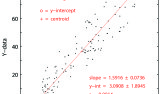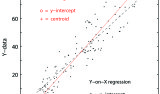Weighted Y-on-XLsqfityw.m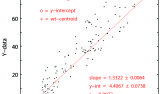Weighted Y-on-XLsqfityz.mMODEL II
REGRESSIONS
M-FILESINGLE LINE PLOTSCOMBINED PLOTS
Major axisLsqfitma.m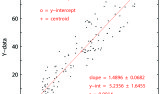Geometric meanLsqfitgm.mLeast-squares-bisectorLsqbisec.m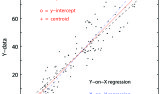Least-squares-cubicLsqcubic.m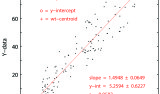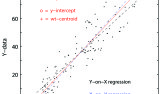#### Footnotes to table:

1. All parameters were calculated with equations from Bevington and Robinson (1992). Their standard deviations for the slope and intercept seem very small when compared to the uncertainties calculated with the other regression equations.
2. Revised weighted model I regression: equations for the slope and intercept were again taken from Bevington and Robinson (1992), however, the standard deviations in these parameters are calculated according to equations derived by York (1966). The uncertainties calculated this way seem more in line with those calculated by the other regressions.
3. The least-squares-bisector algorithm follows the suggestion of Sprent and Dolby (1980) that in the case of model II regressions, an equally strong case can be made for the line that bisects the minor angle between the two model I regressions: Y-on-X and X-on-Y. The uncertainties in the slope and intercept are calculated according to the equations derived by York (1966) in a manner analogous to that used for the geometric mean regression.

• The answers in the table have not been rounded-off to the proper number of significant figures. Instead, I have chosen to list the results obtained with Matlab in standard precision mode so that one can compare these results to those from other programs without an undue emphasis on data truncation and round-off errors.
• Equations for the Y-on-XX-on-Ymajor axisgeometric mean and least-squares-bisectorregressions all run through the centroid: (mean-x,mean-y).
• Likewise, the correlation coefficients for the Y-on-XX-on-Ymajor axis and geometric meanregressions are all the same. Thus, the correlation coefficient is properly interpreted as a measure of the linearity of the data and not how well the line fits the data.
• For the weighted linear regressions and the least squares cubic the correlation coefficients are different owing to the varying weights applied to each data point.

#### References:

• Bevington & Robinson (1992). Data Reduction and Error Analysis for the Physical Sciences, Second Edition, McGraw-Hill, Inc., New York.
• Sprent and Dolby (1980). The geometric mean functional relationship. Biometrics 36: 547-550.
• York (1966). Least-squares fitting of a straight line. Canad. J. Phys. 44: 1079-1086.

For Excel®, MATLAB® and most other commercial programs the inherent line fitting method is the model-I regression.

To determine whether you are using a model-I or a model-II regression

• first find the slope of Y vs X where Y is plotted on the vertical axis and X is plotted on the horizontal axis — this is the “normal” way of doing things. It is also known as the regression of Y-on-X. Call this slope m(y).
• Now reverse X and Y and fit another line; call this slope m(x)’.
• Since X and Y are reversed, we need to find the inverse of m(x)’ to properly compare against m(y), so let m(x) = 1 / m(x)’.
• Now if m(x) = m(y) exactly and r is not equal to 1, then you are using a model-II regression.
• If m(x) is not equal to m(y), then you are using a model-1 regression.

Note that for either model, r^2 = m(y) / m(x). This is known as the Pearson product-moment correlation coefficient. It is a measure of the linearity of the data, not the fit of the line to the data.

To quickly calculate the model-II geometric mean regression slope, m(gm), first determine the model-I regression slope, m(y), and the correlation coefficient, r. The geometric mean slope is then calculated as: m(gm) = m(y) / r. Or, you can use the MATLAB® script file lsqfitgm.

Also note that for datasets where r = 1, m(y) = m(x) = m(gm). In those cases, this test will not tell you which method you are using.